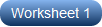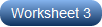# Solving Quadratic Equations by Factoring Worksheets

Catapult to new heights your ability to solve a quadratic equation by factoring, with this assortment of printable worksheets. Backed by three distinct levels of practice, high school students master every important aspect of factoring quadratics. The quadratic equations in these exercise pdfs have real as well as complex roots. Keep to the standard form of a quadratic equation: ax2 + bx + c = 0, where x is the unknown, and a ≠ 0, b, and c are numerical coefficients. Either the given equations are already in this form, or you need to rearrange them to arrive at this form. You can also use algebraic identities at this stage if the equation permits. Once you are here, follow these steps to a tee and you will progress your way to the roots with ease.
Step 1: Find the product of a and c.
Step 2: Determine the two factors of this product that add up to 'b'.
Step 3: Use these factors and rewrite the equation in the factored form.
Step 4: Equate each factor to zero and figure out the roots upon simplification.
Now, you're all set to go! And our free worksheet is sure to give you a flying start!

Triumph in your quadratic equations like never before! The zero-product property signifies that when the product of any two factors is zero, one of the factors must be zero. Implement this rule, and solve the quadratic equations offered in factored form. Set each factor equal to zero, and reach to the roots.Kick-start your quadratic practice with this easy set where each pdf worksheet presents 10 equations with the coefficient of the leading term being 1 in each case. Factorize the constant term in such a way that its factors give the middle-term coefficient when added, and apply the zero-product rule to obtain the real roots.Upgrade your skills with these moderate handouts rendering quadratic equations that have real and imaginary roots. High school students are supposed to rewrite the equation in the standard form and then proceed with the usual factoring and solving steps. Your quadratic learning will now take off!Progress to the next level of difficulty by solving the complicated quadratic equations here! Because they have an expression in place of the unknown, these equations are called disguised quadratic equations. Make an appropriate substitution, convert the equation to general form, and solve for the roots.